# Class 9 Maths notes for Quadrilaterals

Welcome to Class 9 Maths chapter 8 Quadrilaterals notes.The topics in this page are What is Quadrilateral,Parallelogram,Rhombus,Rectangle,square and Mid-point Theorem for Triangles.This is according to CBSE and the NCERT textbook. If you like the study material, feel free to share the link as much as possible.

Table of Content

A quadrilateral is the union of four line-segments determined by four distinct coplanar points of which no three are collinear and the line-segments intersect only at end points.

For ABCD to be quadrilateral, following condition are required
(a) The four points A, B, C and D must be distinct and co-planar.
(b) No three of points A, B, C and D are co-linear.
(c) Line Segments i.e. AB, BC, CD, DA intersect at their end points only.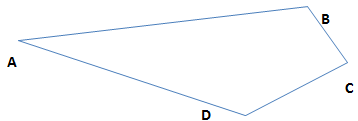1. AB, BC, CD and DA are the four sides.
2. Points $A, B, C \; and \; D$ are the four vertices.
3. $\angle ABC \;, \; \angle BCD \; , \; \angle CDA \; and \; \angle DAC$ are the four angles.
4. AB and CD are the opposite sides.
5. Angle A and C are the opposite angles.
6. AB and BC are the adjacent sides.
7. Angle A and B are the adjacent angles

(1) Sum of all the interior angles is 3600
(2) Sum of all the exterior angles is 3600

A quadrilateral is a four-sided polygon with four angles. There are many kinds of quadrilaterals. The five most common types are the parallelogram, the rectangle, the square, the trapezoid, and the rhombus.

## Parallelogram

A quadrilateral which has both pairs of opposite sides parallel is called a parallelogram.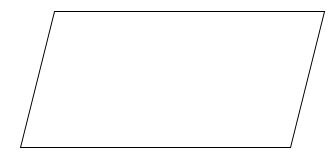Its properties are:
(a)The diagonal of a parallelogram divide into two congruent triangles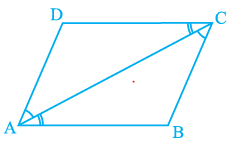In triangle ABC and CDA,
$\angle BCA = \angle DAC$ ( Pair of alternate angles between parallels BC and AD)
$\angle BAC = \angle DCA$ ( Pair of alternate angles between parallels AB and DC)
AC=CA
So, $\Delta ABC \cong \Delta CDA$

(b)The opposite sides of a parallelogram are equal
This can be seen from above proof only. Since it forms two congruent triangle

(c)The opposite angles of a parallelogram are equal
This can be seen from above proof only. Since it forms two congruent triangle
$\angle D= \angle B$ and $\angle A = \angle C$
(d)The diagonals of a parallelogram bisect each other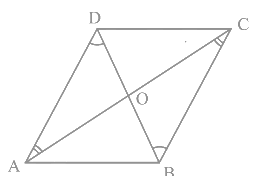In triangle AOD and COB,
$\angle OAD = \angle OCB$ ( Pair of alternate angles between parallels BC and AD)
$\angle ODA = \angle OBC$ ( Pair of alternate angles between parallels AD and BC)
AD=BC So, $\Delta AOD \cong \Delta COB$
So OA=OC and OB=OD, Thus diagonal bisect each other
A quadrilateral is said to a parallelogram if any of these conditions are met
(a) Opposite sides are equal
(b) Opposite angles are equal
(c) Diagonal bisects each other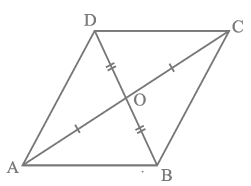We can easily show here $\Delta AOB \cong \Delta COD$ and then $\angle ABO = \angle CDO$, By alternate angle theorem, AB||CD and hence parallelogram
(d) A pair of opposite are parallel and equal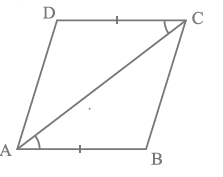We can easily show here $\Delta ABC \cong \Delta CDA$ and then BC || AD and hence parallelogram

## Trapezium

A quadrilateral which has one pair of opposite sides parallel is called a trapezium.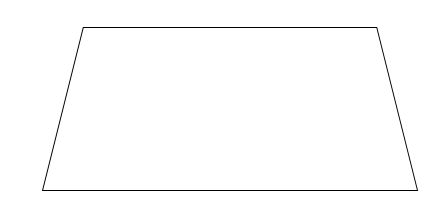## Rhombus

Rhombus is a parallelogram in which any pair of adjacent sides is equal.
Properties of a rhombus:
(a)All sides of a rhombus are equal
(b)The opposite angles of a rhombus are equal
(c)The diagonals of a rhombus bisect each other at right angles.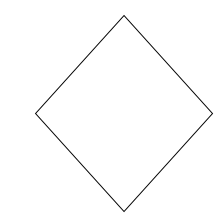## Rectangle

A parallelogram which has one of its angles a right angle is called a rectangle.
Properties of a rectangle are:
(a)The opposite sides of a rectangle are equal
(b) Each angle of a rectangle is a right-angle.
(c) The diagonals of a rectangle are equal.
(d) The diagonals of a rectangle bisect each other.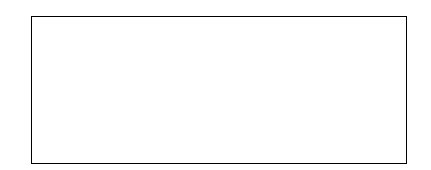## Square

A quadrilateral, all of whose sides are equal and all of whose angles are right angles.
Properties of square are:
(a)All the sides of a square are equal.
(b) Each of the angles measures $90^0$.
(c) The diagonals of a square bisect each other at right angles.
(d) The diagonals of a square are equal.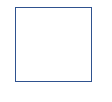All the quadrilaterals can be shown in Venn diagram like this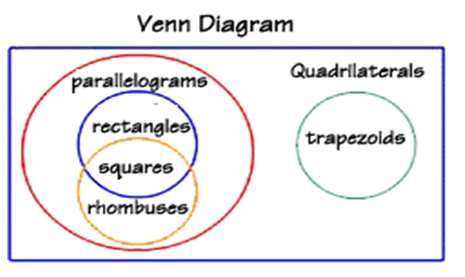We can divide the entire set of quadrilateral in three major parts
(2)parallelograms
(3) trapezoids.
Some Other observation from this
(a) A square is always a parallelogram Similarly a rectangle is always a parallelogram
(b) A square is always a rectangle,rhombus
(c) A rhombus can be square.
(d) A rectangle has four right angles.
(e) A rectangle is not always a rhombus
(f) A Trapezium is not a parallelogram

## Mid-point Theorem for Triangles

Theorem-I
The line segment joining the mid points of the two sides of the triangle is parallel to the third side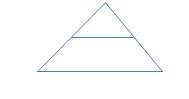Theorem-II
A line drawn through mid point of one side of a triangle and parallel to another side bisect the third side of the triangle

## How to solve the angle Problem in Quadrilateral

1. We will be given problem where three angles of quadrilateral known or two angles are known with other two angles having some relationship
2. Case1 : Three angles are known,How to find the fourth angle

As per angle property of quadrilateral
Sum of all the interior angles is $360^0$ So
$A+B+C+D=360$
If A,B,C are known we can find D easily

3. case 2: two angles are known with other two angles having some relationship
A and B are known and $2C-D= 50$
Now again we know that
$A+B+C+D=360$
Then C+D=
We can find the values of C and D from below two equations
2C-D= 50
C+D=
Example 1:
The angles of quadrilateral are in the ratio 3 : 5 : 9 : 13. Find all the angles of the quadrilateral
Solution:
Angles are 3x,5x,9x,13x
So
$3x+5x+9x+13x=360$
$30x=360$
x=12

So angles are
36, 60,108, 156

Example 2
AX and CY are respectively the bisectors of the opposite angles A and C of a parallelogram ABCD.
Show that AX || C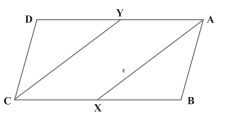Solution:
Now $\angle A = \angle C$
So $\angle YAX=\angle YCX$
Also, $\angle AYC + \angle YCX = 180^0$ (Because YA || CX)
Therefore, $\angle AYC + \angle YAX = 180^0$
So, AX || CY (As interior angles on the same side of the transversal are supplementary)

2. A quadrilateral is also sometimes called Quadrangle ("four angles"), so it sounds like "triangle"
or Tetragon ("four and polygon"), so it sounds like "pentagon", "hexagon", etc.
3. Quadrilaterals can be classified in terms of parallel pair of lines also
No Pair of Parallel lines: General quadrilaterals
One pair of parallel lines: Trapezium
Two pair of parallel lines : square,parallelogram,rectangle,rhombus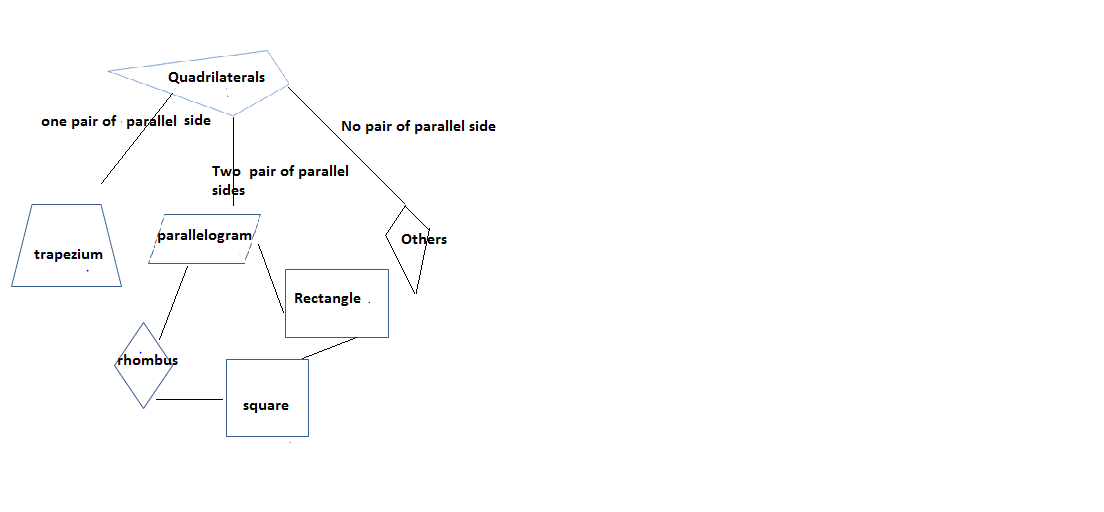4. Quadrilaterals can be classified in terms of congruent sides also
All sides congruent: Square,rhombus
One pair of sides are congruent:Isococeles Trapezium
Two pair of sides are congruent: Rectangle,parallelogram,Kite

### Quiz Time

Question 1 What is the sum of the angle of quadrilaterals ?
A. $180^0$
B. $270^0$
C. $360^0$
D. $90^0$
Question 2 Which is of these is not of parallelogram ?
A. Diagonal bisect each other
B. Opposite angle are equal
C. Opposite sides are equal
D. opposite angles are bisected by the diagonals
Question 3 : Angles of a quadrilateral are in the ratio 3 : 5 : 5 : 7. Find all the angles of the quadrilateral.
A. $54^0,90^0,90^0,126^0$
B. $54^0,80^0,80^0,146^0$
C. $34^0,100^0,100^0,126^0$
D. None of these
Question 4 Opposite angles of a quadrilateral PQRS are equal. If PQ = 10 cm, What is the value of RS
A. 10
B. 5
C. 20
D. Insufficient details
Question 5 which of the following is true?
A. A quadrilateral has four sides
B. A quadrilateral has three diagonals
C. A quadrilaterals has four angles
D. None of these
Question 6 ABCD and AEFG are two parallelograms. If $\angle C = 61^0$, determine $\angle G$.?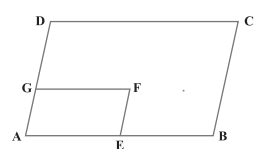A. $61^0$
B. $119^0$
C. $90^0$
D. None of these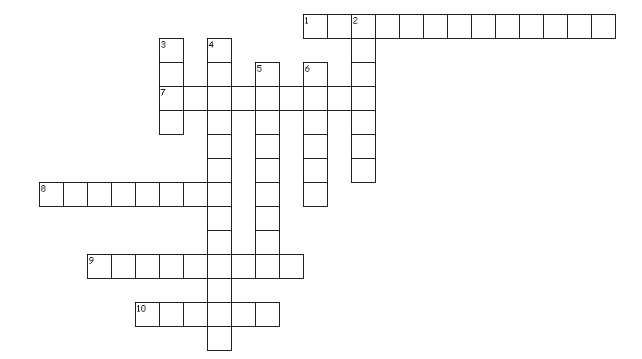Across
1. Quadrilaterals where both pair of opposite sides are parallel
7. Quadrilaterals where one pair opposite sides are parallel
8. Line joining the opposite vertices in quadrilaterals
9. A triangle where two sides are equal
10. A parallelogram where all the sides are equal and angle $90^0$
Down
2. A parallelogram where all the sides are equal
4. A polygon of four sides
5. A parallelogram where opposite sides are equal angle $90^0$
6. The diagonals .......in the parallelogram

## Summary

Here is the quadrilaterals class 9 notes Summary
• A quadrilateral is a four-sided polygon with four angles and sum of the angle is 360°
• A quadrilateral which has both pairs of opposite sides parallel is called a parallelogram.In a parallelogram opposite sides and angles are equal. Diagonal bisect each other
• Rectangle is a parallelogram which has one of its angles a right angle .Diagonal bisect each other and are equal
• The line segment joining the mid points of the two sides of the triangle is parallel to the third side